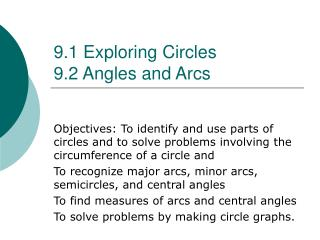# 9.1 Exploring Circles 9.2 Angles and Arcs - PowerPoint PPT PresentationDownload Presentation9.1 Exploring Circles 9.2 Angles and Arcs

9.1 Exploring Circles 9.2 Angles and ArcsDownload Presentation## 9.1 Exploring Circles 9.2 Angles and Arcs

- - - - - - - - - - - - - - - - - - - - - - - - - - - E N D - - - - - - - - - - - - - - - - - - - - - - - - - - -
##### Presentation Transcript

1. 9.1 Exploring Circles9.2 Angles and Arcs Objectives: To identify and use parts of circles and to solve problems involving the circumference of a circle and To recognize major arcs, minor arcs, semicircles, and central angles To find measures of arcs and central angles To solve problems by making circle graphs.

2. DEFINITIONS • circle– • circumference– • diameter – • radius–

3. Definition • Chord –

4. DEFINITIONS (Cont) • Minor arc– • Major arc – • Semicircle – • Adjacent Arcs –

5. DEFINITIONS (Cont) • sector – • central angle – • Concentric circles –

6. CIRCUMFERENCE • The circumference of a circle is equal to  times the diameter or 2 times the radius C = d or C = 2r • Find the circumference of a circle whose diameter is 3 ft. Round your answer to three significant digits

7. Y 110 110 O Z Arc Measure The measure of a center angle is equal to the measure of the intercepted arc. Center Angle Intercepted Arc Give is the diameter, find the value of x and y and z in the figure. Example:

8. Find the measure of each arc. • BC = • BD = • ABC = • AB =

9. Ex. 1: Finding Measures of Arcs • Find the measure of each arc of R. 80°

10. Note: • Two arcs of the same circle are adjacent if they intersect at exactly one point. You can add the measures of adjacent areas. • Arc Addition Postulate. The measure of an arc formed by two adjacent arcs is the sum of the measures of the two arcs. m = m + m

11. Ex. 2: Finding Measures of Arcs • Find the measure of each arc. 40° 80° 110°

12. Example: Find the measure of each arc.

13. ARC LENGTH • The length of an arc equals the ratio of the number of degrees of the arc to 360° times the circumference • Determine the length of a 30° arc on a circle with a radius of 5 m:

14. POSTULATE EXAMPLE Determine the length of arc AB in the figure below given that CD is 24 cm, COD = 92, and AOB = 58 C D  O A B 14

15. PRACTICE PROBLEMS (Cont) Determine the circumference of a circle with a radius of 2.5 inches. Determine the arc length of a circle with a 5 m radius and a 50° arc. 15

16. Congruent Arcs • Two arcs which have the same measure are not necessarily congruent arcs. • Congruent arcs have the same measure and are parts of the same circle or congruent circles.

17. Relating Congruent Arcs, Chords & Central Angles • Theorem: If two angles of a circle (or of congruent circles) are congruent, then their intercepted arcs are congruent. • Theorem: If two arcs are congruent, then their central angles are congruent.

18. Circles that have a common center are called concentric circles. Concentric circles No points of intersection Concentric circles

19. Here is a circle graph that shows how people really spend their time. Find the measure of each central angle in degrees. • Sleep • Food • Work • Must Do • Entertainment • Other